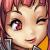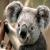## 大家看看题目，和答案对吗？

javacc 发布于 2013/01/10 09:06

为什么80%的码农都做不了架构师？->>>题目：判断101-200之间有多少个素数，并输出所有素数。

1.程序分析：判断素数的方法：用一个数分别去除2sqrt(这个数)，如果能被整除，

public class exp2{

public static void main(String args[]){

int i=0;

math mymath = new math();

for(i=2;i<=200;i++)

if(mymath.iszhishu(i)==true)

System.out.println(i);

}

}

class math

{

public int f(int x)

{

if(x==1 || x==2)

return 1;

else

return f(x-1)+f(x-2);

}

public boolean iszhishu(int x)

{

for(int i=2;i<=x/2;i++)

if (x % 2==0 )

return false;

return true;

}

}

00
0#### 引用来自“紅顏為君笑”的答案